## Selina Concise Mathematics Class 7 ICSE Solutions Chapter 19 Congruency: Congruent Triangles

Selina Publishers Concise Mathematics Class 7 ICSE Solutions Chapter 19 Congruency: Congruent Triangles

### Congruency: Congruent Triangles Exercise 19 – Selina Concise Mathematics Class 7 ICSE Solutions

Question 1.
State, whether the pairs of triangles given in the following figures are congruent or not: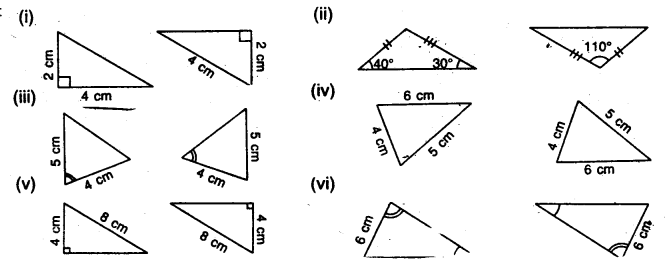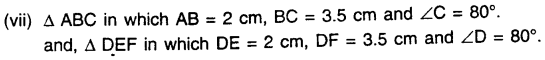Solution:Question 2.
In the given figure, prove that:
∆ABD ≅ ∆ ACD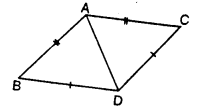Solution: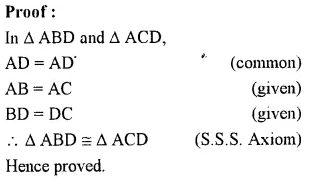Question 3.
Prove that:
(ii) ∠B = ∠D
(iii) AC bisects angle DCB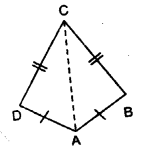Solution:Question 4.
Prove that:
(i) ∆ABD  ≡ ∆ACD
(ii) ∠B = ∠CSolution:Question 5.
In the given figure, prove that:
(i) ∆ACB ≅ ∆ECD
(ii) AB = EDSolution: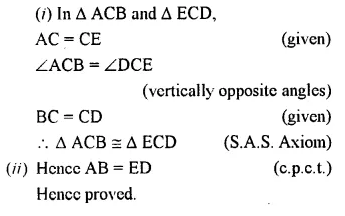Question 6.
Prove that:
(i) ∆ ABC ≅ ∆ ADC
(ii) ∠B = ∠D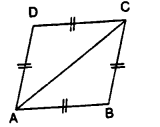Solution:Question 7.
In the given figure, prove that: BD = BC.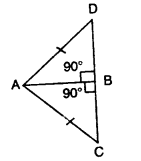Solution: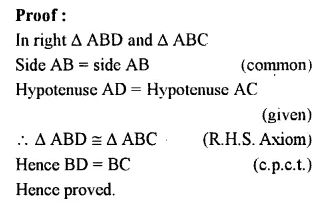Question 8.
In the given figure ;
∠1 = ∠2 and AB = AC. Prove that:
(i) ∠B = ∠C
(ii) BD = DC
(iii) AD is perpendicular to BC.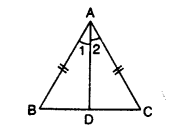Solution: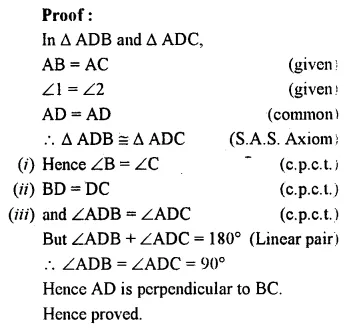Question 9.
In the given figure prove tlyat:
(i) PQ = RS
(ii) PS = QRSolution:Question 10.
(i) ∆ XYZ ≅ ∆ XPZ
(ii) YZ = PZ
(iii) ∠YXZ = ∠PXZSolution:Question 11.
In the given figure, prove that:
(i) ∆ABC ≅ ∆ DCB
(ii) AC=DBSolution: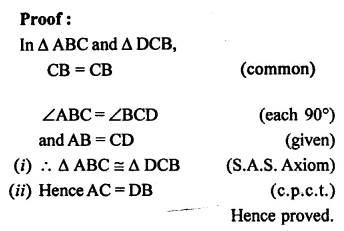Question 12.
In the given figure, prove that:
(i) ∆ AOD ≅ ∆ BOCSolution: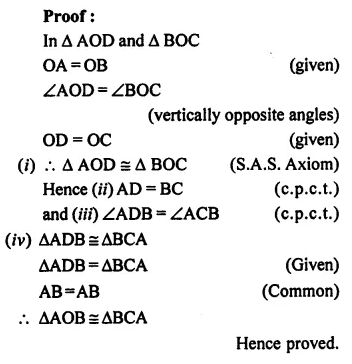Question 13.
ABC is an equilateral triangle, AD and BE are perpendiculars to BC and AC respectively. Prove that:
(ii)BD = CE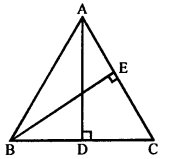Solution: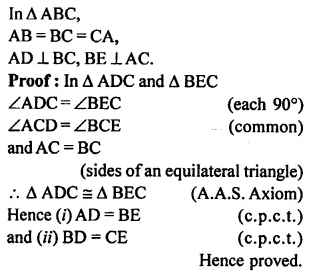Question 14.
Use the informations given in the following figure to prove triangles ABD and CBD are congruent.
Also, find the values of x and y.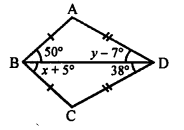Solution: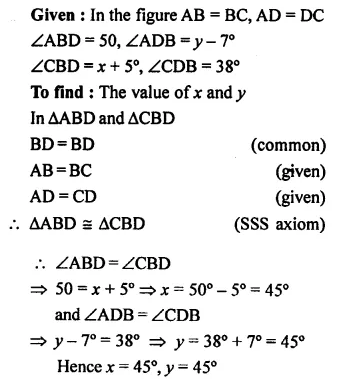Question 15.
The given figure shows a triangle ABC in which AD is perpendicular to side BC and BD = CD. Prove that:
(i) ∆ABD ≅ ∆ACD
(ii) AB=AC
(iii) ∠B = ∠C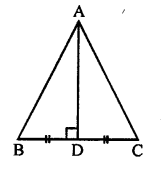Solution: